# RD Sharma Solutions For Class 7 Maths Chapter 2 Fractions Exercise 2.3

RD Sharma Solutions for Class 7 Maths Chapter 2 – Fractions Exercise 2.3 is the best study material for those students who are finding difficulties in solving problems. Our specialist teachers formulate these exercises to help you with your exam preparation to achieve good marks in Maths. Students can download the PDF of these solutions from the given links. By practising RD Sharma Solutions for Class 7, students will be able to grasp the concepts perfectly. It also helps in boosting their confidence, which plays a pivotal role in the examinations.

## Download the PDF of RD Sharma Solutions For Class 7 Maths Chapter 2 – Fractions Exercise 2.3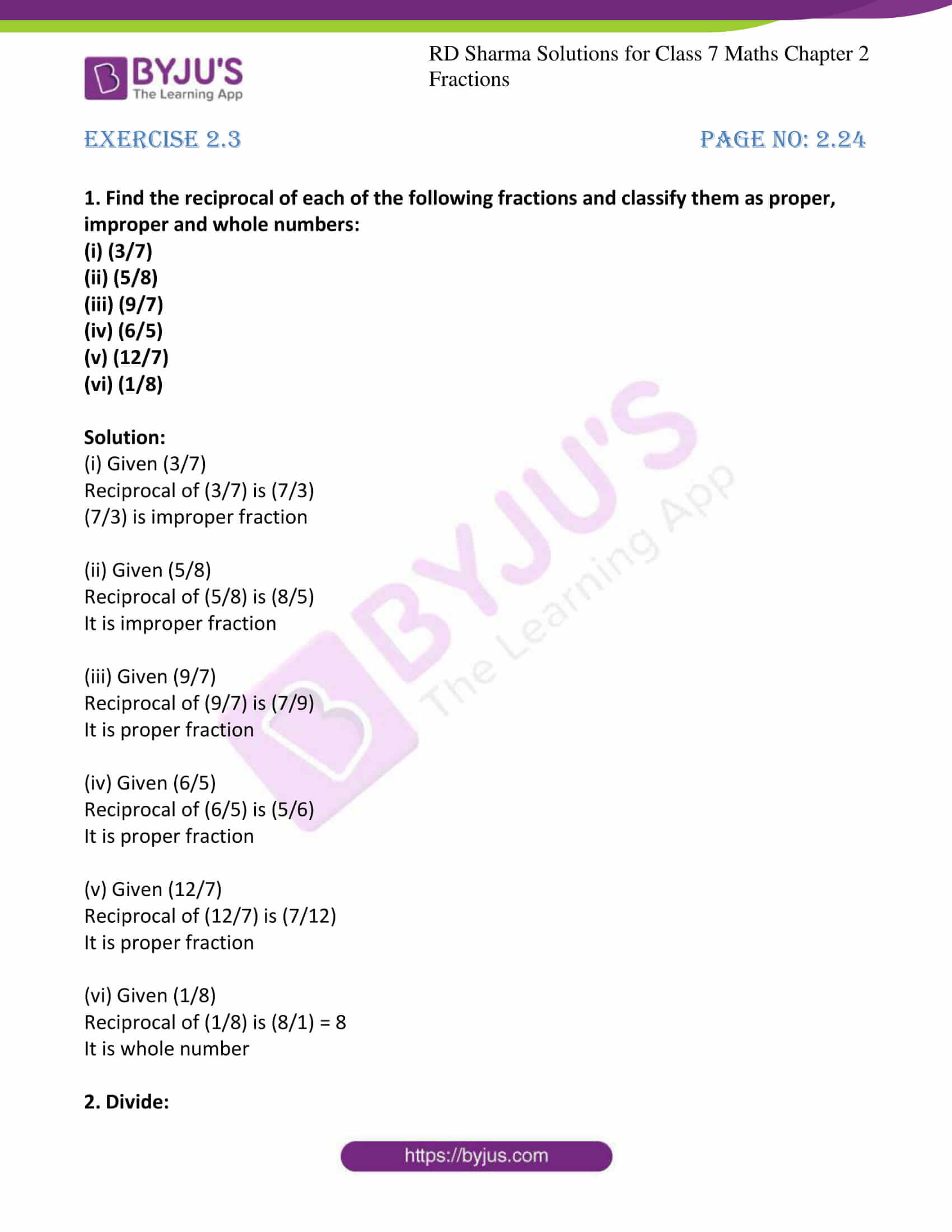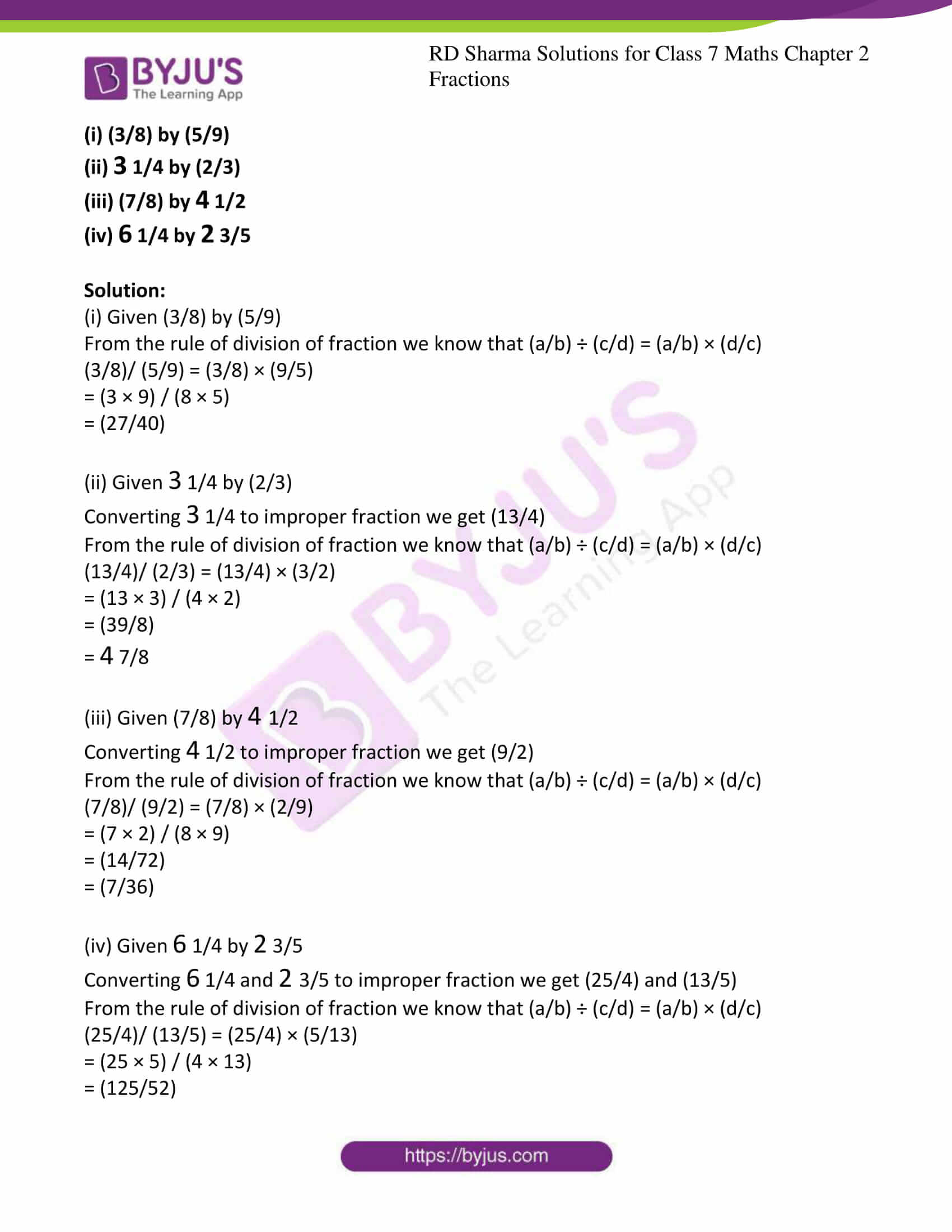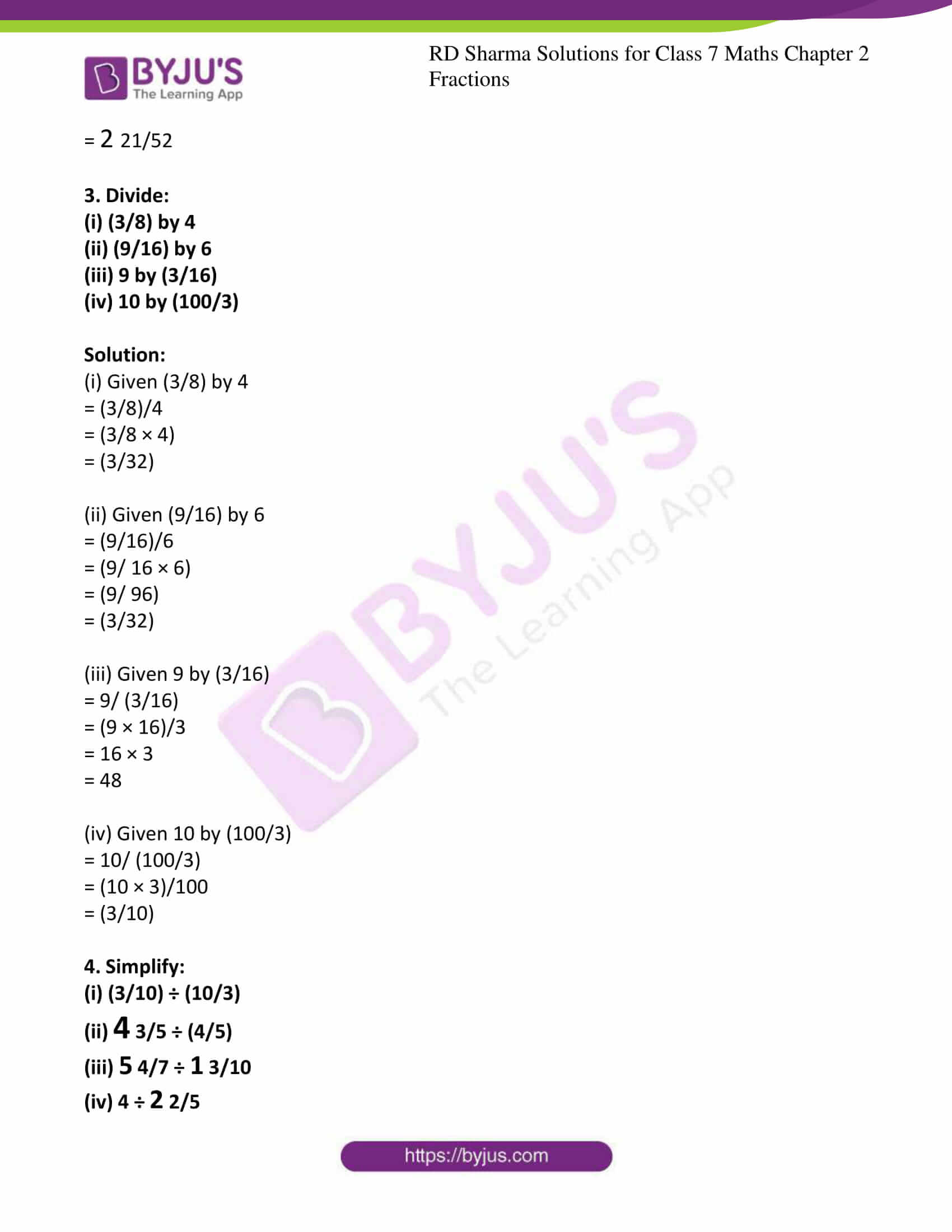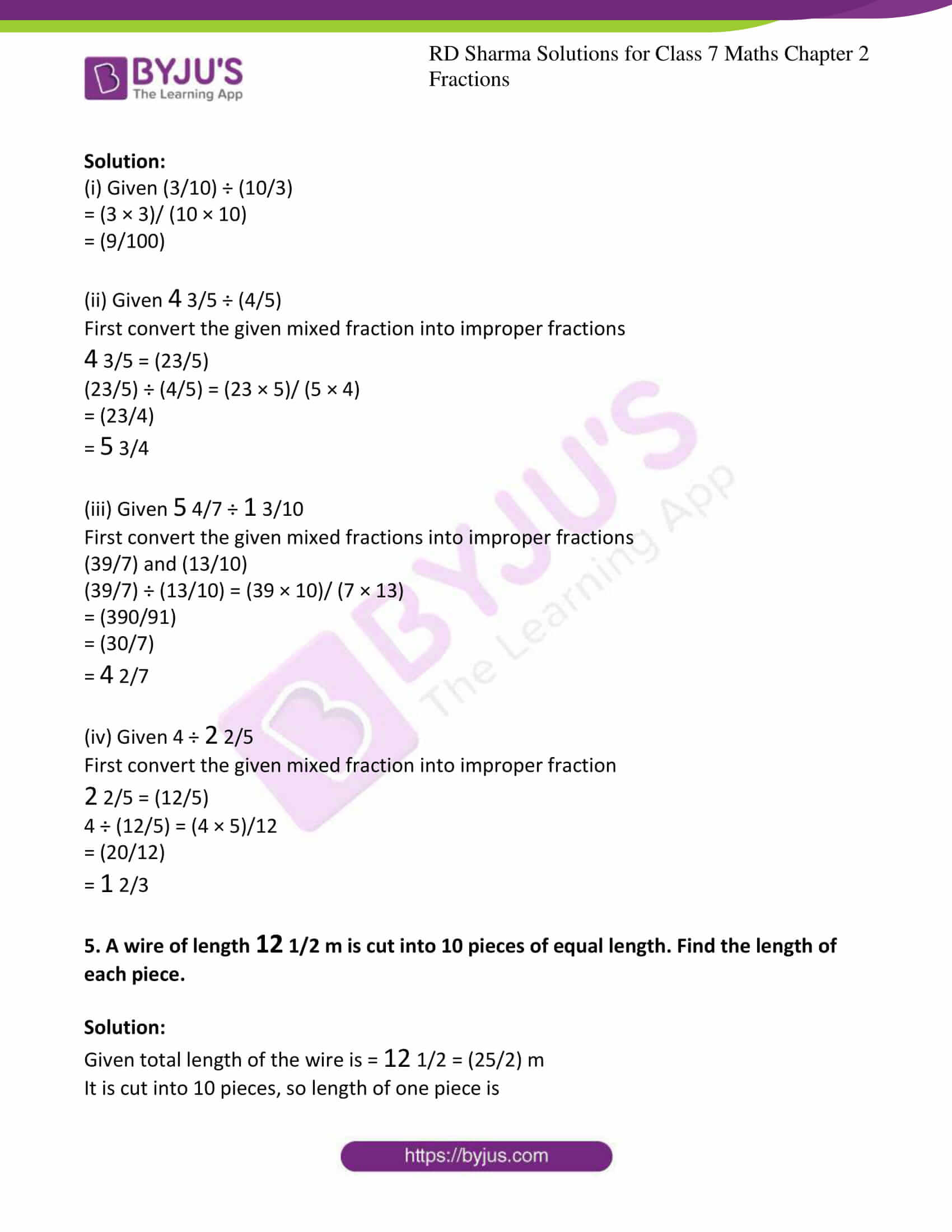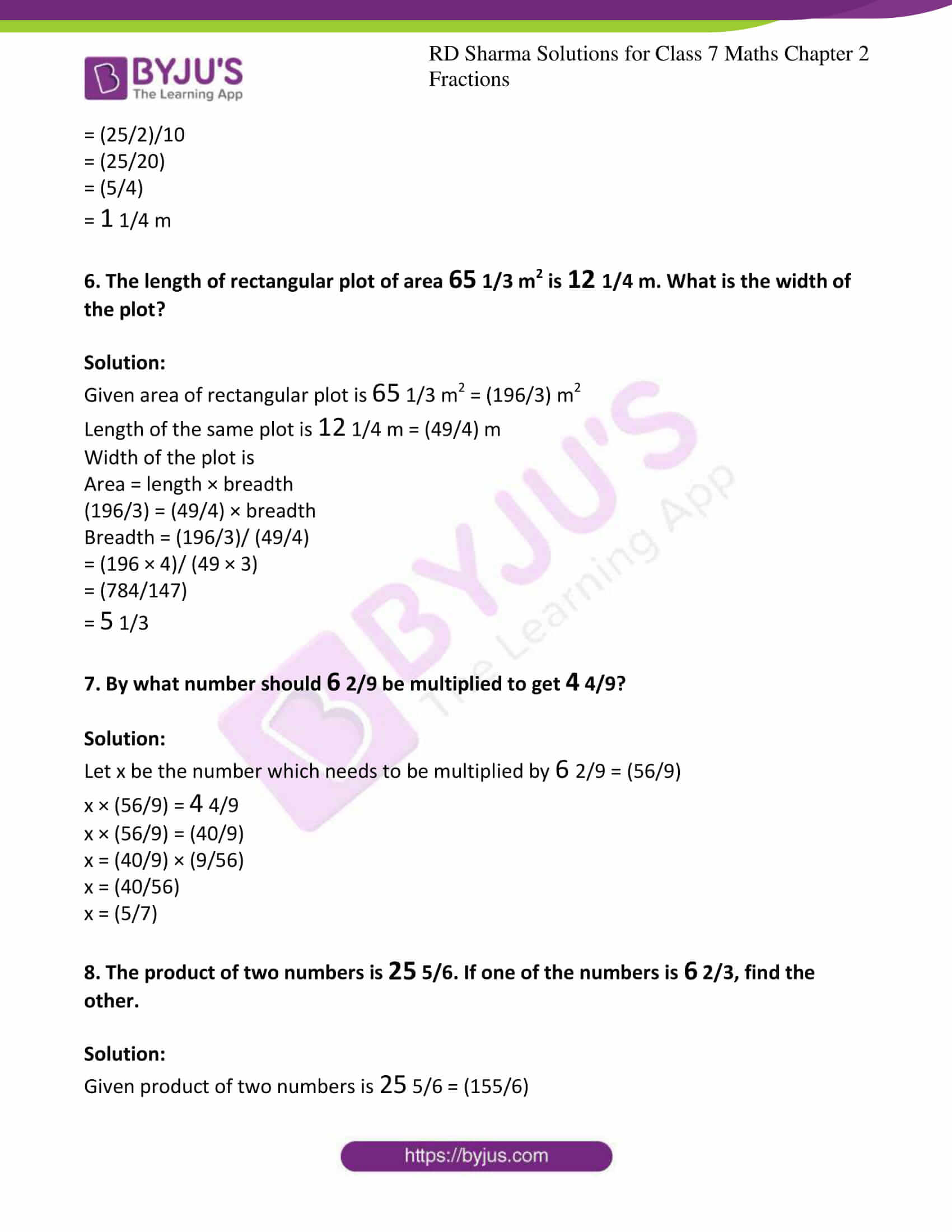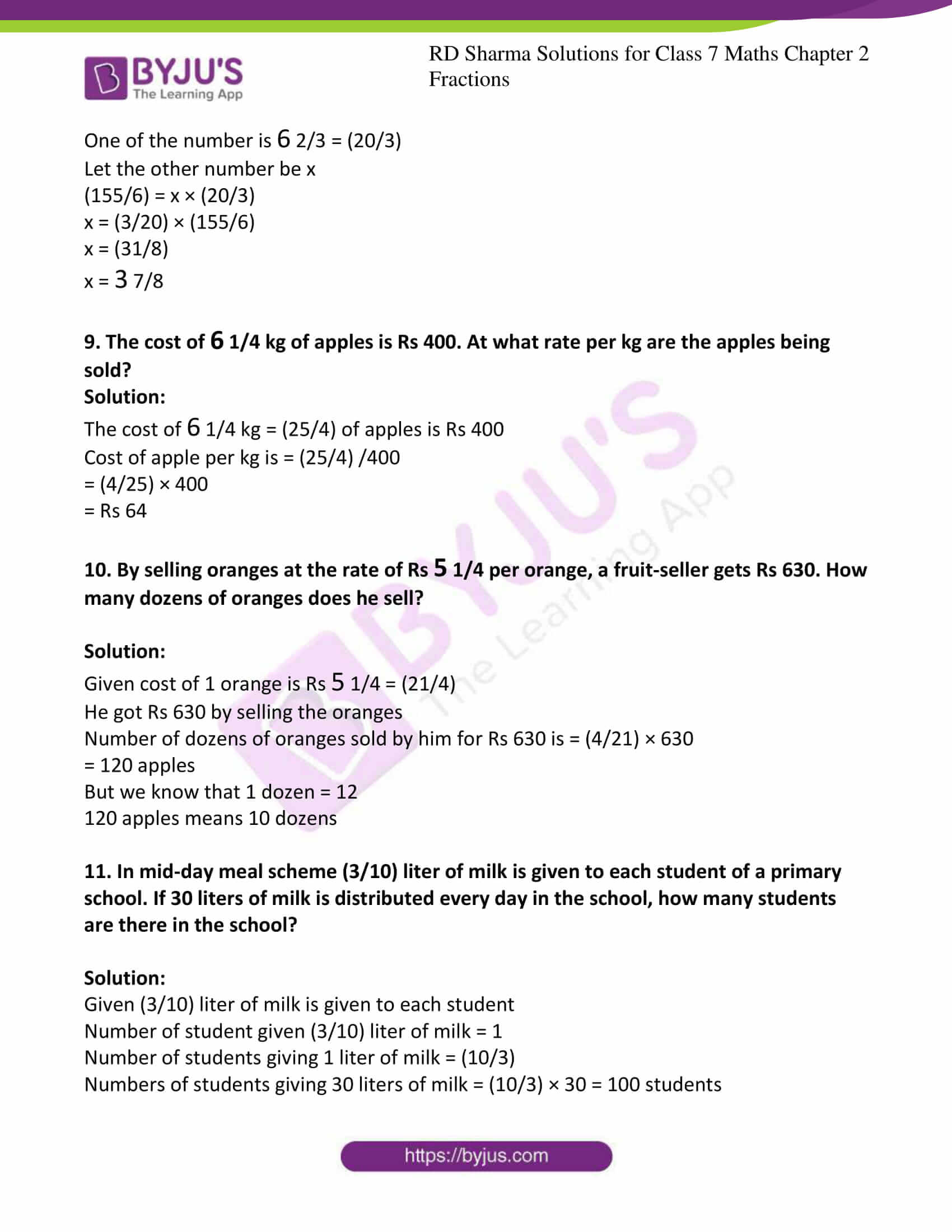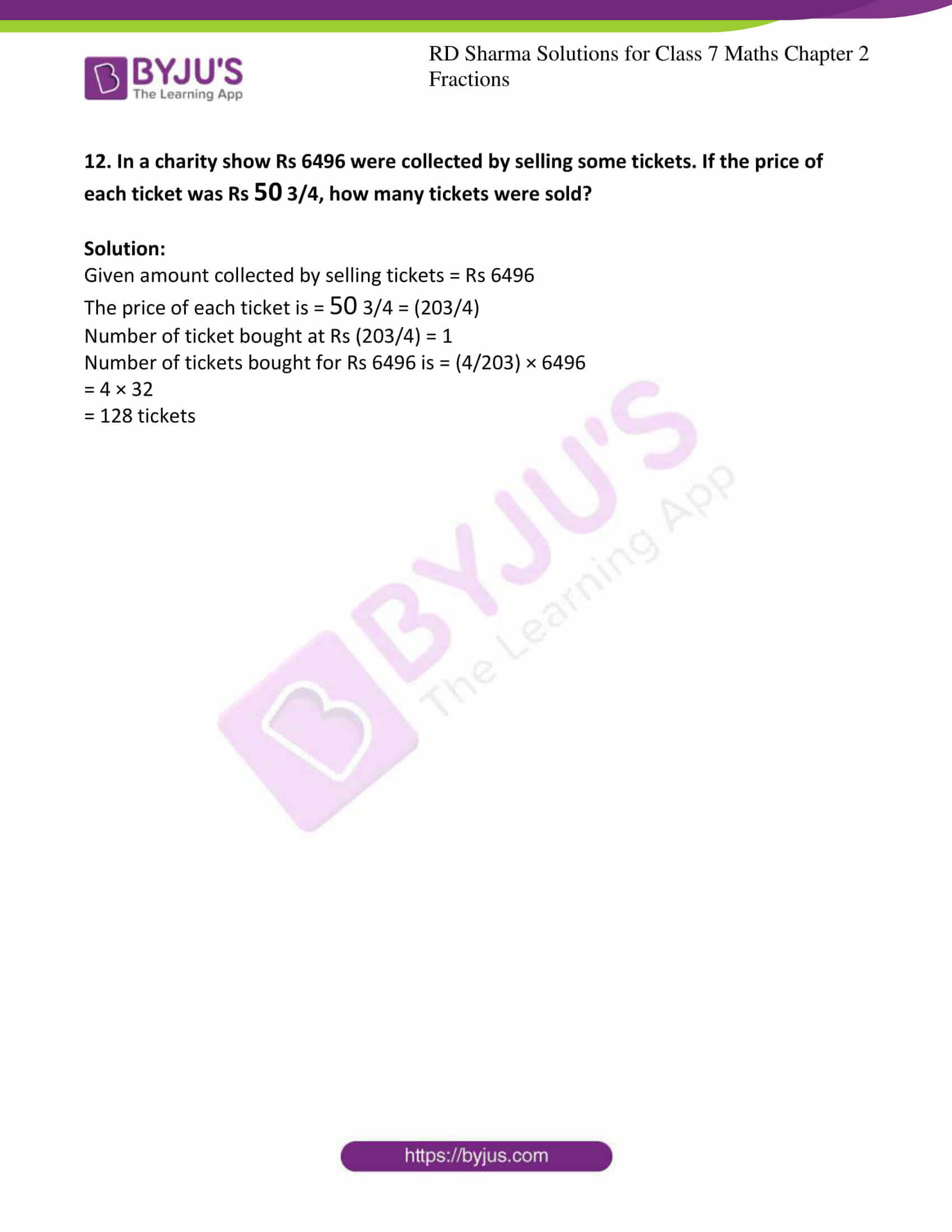### Access answers to Maths RD Sharma Solutions For Class 7 Chapter 2 – Fractions Exercise 2.3

Exercise 2.3 Page No: 2.24

1. Find the reciprocal of each of the following fractions and classify them as proper, improper and whole numbers:

(i) (3/7)

(ii) (5/8)

(iii) (9/7)

(iv) (6/5)

(v) (12/7)

(vi) (1/8)

Solution:

(i) Given (3/7)

Reciprocal of (3/7) is (7/3)

(7/3) is improper fraction

(ii) Given (5/8)

Reciprocal of (5/8) is (8/5)

It is improper fraction

(iii) Given (9/7)

Reciprocal of (9/7) is (7/9)

It is proper fraction

(iv) Given (6/5)

Reciprocal of (6/5) is (5/6)

It is proper fraction

(v) Given (12/7)

Reciprocal of (12/7) is (7/12)

It is proper fraction

(vi) Given (1/8)

Reciprocal of (1/8) is (8/1) = 8

It is whole number

2. Divide:

(i) (3/8) by (5/9)

(ii) 3 1/4 by (2/3)

(iii) (7/8) by 4 1/2

(iv) 6 1/4 by 2 3/5

Solution:

(i) Given (3/8) by (5/9)

From the rule of division of fraction we know that (a/b) ÷ (c/d) = (a/b) × (d/c)

(3/8)/ (5/9) = (3/8) × (9/5)

= (3 × 9) / (8 × 5)

= (27/40)

(ii) Given 3 1/4 by (2/3)

Converting 3 1/4 to improper fraction we get (13/4)

From the rule of division of fraction we know that (a/b) ÷ (c/d) = (a/b) × (d/c)

(13/4)/ (2/3) = (13/4) × (3/2)

= (13 × 3) / (4 × 2)

= (39/8)

= 4 7/8

(iii) Given (7/8) by 4 1/2

Converting 4 1/2 to improper fraction we get (9/2)

From the rule of division of fraction we know that (a/b) ÷ (c/d) = (a/b) × (d/c)

(7/8)/ (9/2) = (7/8) × (2/9)

= (7 × 2) / (8 × 9)

= (14/72)

= (7/36)

(iv) Given 6 1/4 by 2 3/5

Converting 6 1/4 and 2 3/5 to improper fraction we get (25/4) and (13/5)

From the rule of division of fraction we know that (a/b) ÷ (c/d) = (a/b) × (d/c)

(25/4)/ (13/5) = (25/4) × (5/13)

= (25 × 5) / (4 × 13)

= (125/52)

= 2 21/52

3. Divide:

(i) (3/8) by 4

(ii) (9/16) by 6

(iii) 9 by (3/16)

(iv) 10 by (100/3)

Solution:

(i) Given (3/8) by 4

= (3/8)/4

= (3/8 × 4)

= (3/32)

(ii) Given (9/16) by 6

= (9/16)/6

= (9/ 16 × 6)

= (9/ 96)

= (3/32)

(iii) Given 9 by (3/16)

= 9/ (3/16)

= (9 × 16)/3

= 16 × 3

= 48

(iv) Given 10 by (100/3)

= 10/ (100/3)

= (10 × 3)/100

= (3/10)

4. Simplify:

(i) (3/10) ÷ (10/3)

(ii) 4 3/5 ÷ (4/5)

(iii) 5 4/7 ÷ 1 3/10

(iv) 4 ÷ 2 2/5

Solution:

(i) Given (3/10) ÷ (10/3)

= (3 × 3)/ (10 × 10)

= (9/100)

(ii) Given 4 3/5 ÷ (4/5)

First convert the given mixed fraction into improper fractions

4 3/5 = (23/5)

(23/5) ÷ (4/5) = (23 × 5)/ (5 × 4)

= (23/4)

= 5 3/4

(iii) Given 5 4/7 ÷ 1 3/10

First convert the given mixed fractions into improper fractions

(39/7) and (13/10)

(39/7) ÷ (13/10) = (39 × 10)/ (7 × 13)

= (390/91)

= (30/7)

= 4 2/7

(iv) Given 4 ÷ 2 2/5

First convert the given mixed fraction into improper fraction

2 2/5 = (12/5)

4 ÷ (12/5) = (4 × 5)/12

= (20/12)

= 1 2/3

5. A wire of length 12 1/2 m is cut into 10 pieces of equal length. Find the length of each piece.

Solution:

Given total length of the wire is = 12 1/2 = (25/2) m

It is cut into 10 pieces, so length of one piece is

= (25/2)/10

= (25/20)

= (5/4)

= 1 1/4 m

6. The length of rectangular plot of area 65 1/3 m2 is 12 1/4 m. What is the width of the plot?

Solution:

Given area of rectangular plot is 65 1/3 m2 = (196/3) m2

Length of the same plot is 12 1/4 m = (49/4) m

Width of the plot is

= (196 × 4)/ (49 × 3)

= (784/147)

= 5 1/3

7. By what number should 6 2/9 be multiplied to get 4 4/9?

Solution:

Let x be the number which needs to be multiplied by 6 2/9 = (56/9)

x × (56/9) = 4 4/9

x × (56/9) = (40/9)

x = (40/9) × (9/56)

x = (40/56)

x = (5/7)

8. The product of two numbers is 25 5/6. If one of the numbers is 6 2/3, find the other.

Solution:

Given product of two numbers is 25 5/6 = (155/6)

One of the number is 6 2/3 = (20/3)

Let the other number be x

(155/6) = x × (20/3)

x = (3/20) × (155/6)

x = (31/8)

x = 3 7/8

9. The cost of 6 1/4 kg of apples is Rs 400. At what rate per kg are the apples being sold?

Solution:

The cost of 6 1/4 kg = (25/4) of apples is Rs 400

Cost of apple per kg is = (25/4) /400

= (4/25) × 400

= Rs 64

10. By selling oranges at the rate of Rs 5 1/4 per orange, a fruit-seller gets Rs 630. How many dozens of oranges does he sell?

Solution:

Given cost of 1 orange is Rs 5 1/4 = (21/4)

He got Rs 630 by selling the oranges

Number of dozens of oranges sold by him for Rs 630 is = (4/21) × 630

= 120 apples

But we know that 1 dozen = 12

120 apples means 10 dozens

11. In mid-day meal scheme (3/10) liter of milk is given to each student of a primary school. If 30 liters of milk is distributed every day in the school, how many students are there in the school?

Solution:

Given (3/10) liter of milk is given to each student

Number of student given (3/10) liter of milk = 1

Number of students giving 1 liter of milk = (10/3)

Numbers of students giving 30 liters of milk = (10/3) × 30 = 100 students

12. In a charity show Rs 6496 were collected by selling some tickets. If the price of each ticket was Rs 50 3/4, how many tickets were sold?

Solution:

Given amount collected by selling tickets = Rs 6496

The price of each ticket is = 50 3/4 = (203/4)

Number of ticket bought at Rs (203/4) = 1

Number of tickets bought for Rs 6496 is = (4/203) × 6496

= 4 × 32

= 128 tickets

## RD Sharma Solutions for Class 7 Maths Chapter – 2 Fractions Exercise 2.3

Exercise 2.3 of RD Sharma Solutions for Class 7 Maths Chapter 2 Fractions explains the fundamental operations on fractions. Some of the topics prior to exercise 2.3 are listed below.

• Reciprocal of fractions
• Division of fractions

This exercise can be used as a model of reference by the students to improve their conceptual knowledge and understand the different ways used to solve the problems.